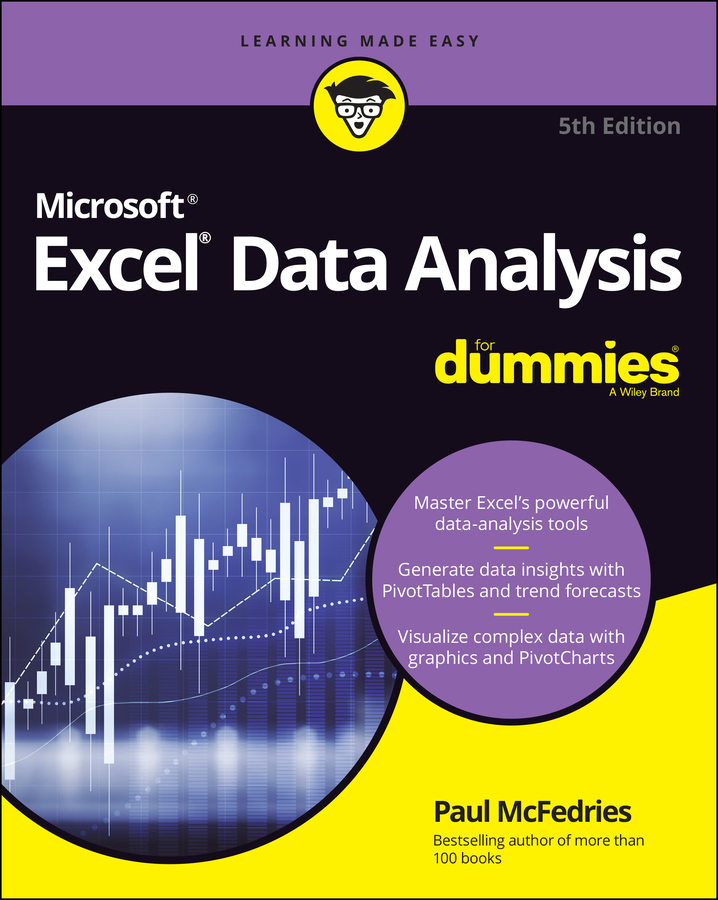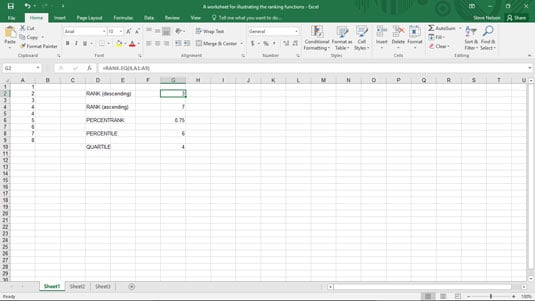##### Excel Data Analysis For DummiesThe QUARTILE.EXC and QUARTILE.INC functions in Excel determine the array value at a specified quartile in an array—and work in a manner similar to the percentile functions.

The QUARTILE.EXC formula uses the syntax

`=QUARTILE.EXC(<i>array</i>,quart)`

The QUARTILE.INC formula uses the syntax

`=QUARTILE.INC(<i>array</i>,<i>quart</i>)`

where array gives the array of values and quart gives the quartile of the value that you want to find.

To find the value in the first quartile in the array of values (inclusive) shown in the worksheet range A1:A9 in this figure, use the formula4." width="535"/>
The function returns the value 4.
`=QUARTILE.INC(A1:A9,2)`

The function returns the value 4, because the value 4 is at the 50th percentile in this array. This formula appears in cell G10 in the worksheet shown.

To find the value in the first quartile in the array of values (exclusive) shown in the worksheet range A1:A9 in the figure, use the formula

`=QUARTILE.EXC(A1:A9,2)`

The function also returns the value 4, because the value 4 is also at the 50th percentile in this array.